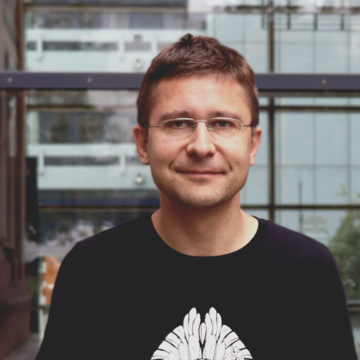# PD Dr. Sebastian Klein

### Research Interest: Integrable Systems

Kontakt

In general. One of my two main research interests is in (infinite-dimensional) integrable systems associated to partial differential equations, their spectral theory and their relations to geometry.

The sinh-Gordon equation. One such integrable system that I have studied extensively is that of the sinh-Gordon equation. This equation is of geometric relevance, because every real-valued two-dimensional solution of this equation corresponds to a minimal surface in the 3-sphere or to a constant mean curvature (cmc) surface in the Euclidean 3-space without umbilic points. Beginning from the case where the solution is doubly periodic (which was investigated by Hitchin and by Pinkall/Sterling, and which corresponds to minimal resp. cmc tori) I investigated simply periodic solutions. I adapted Hitchin's method of constructing spectral data for doubly-periodic solutions to the simply-periodic case, and found asymptotic characterizations for spectral data of this kind. I was also able to solve the inverse problem associated to this situation, i.e. to reconstruct the solution of the sinh-Gordon equation from its spectral data.

For the future I plan to extend this research to investigate solutions of the sinh-Gordon equation which have isolated singularities. Such solutions with isolated singularities arise from minimal surfaces resp. cmc surfaces with umbilic points. It is highly interesting to consider such solutions because compact minimal resp. cmc surfaces of genus g at least 2 always have umbilic points (3g-3 of them, when counted with multiplicity). Presently, very little is known about the geometry and moduli space of them, and understanding their geometry near the umbilic points (corresponding to solutions of the sinh-Gordon equation with isolated singularities), it might be possible to make a step towards understanding the moduli space of these surfaces

The non-linear Schrödinger equation. Another interesting integrable system is the one associated to the (self-focusin) non-linear Schrödinger (NLS) equation. This partial differential equation is of course originally motivated by its significance in quantum mechanics, but another inner-mathematical motivation is that it describes closed curves in the 3-dimensional space forms and their binormal flow. One important result is that closed finite gap curves are dense in the set of all closed curves. For R^3, this result has been shown earlier by Grinevich (by other methods); by applying integrable systems methods, I have extended this result in joint work with Martin Kilian to S^3, H^3 and also to the 2-dimensional space forms.

Pinkall/Sterling have conjectured that for any closed curve in a 2- or 3-dimensional space form, there exists a sequence of cmc tori which converges to the given curve in a certain sense; this is called the soul conjecture. I am interested in applying methods of integrable systems to this conjecture. Because closed curves correspond to the integrable system of the NLS equation, whereas cmc tori correspond to finite type solutions of the sinh-Gordon equation, the interrelations between these two integrable systems are of fundamental importance to this approach.

Publications.

### Research Interest: The geometry of Riemannian symmetric spaces

My other main research interest is in the geometry of Riemannian symmetric spaces, in particular in the geometry of submanifolds which satisfy a curvature condition. The simplest submanifolds of a Riemannian manifold are the totally geodesic ones (i.e. those with vanishing second fundamental form). In spite of their simplicity, the classification of the totally geodesic submanifolds already poses a significant problem. I have classified the totally geodesic submanifolds in the complex quadric, which is a Riemannian symemtric space of rank 2 (one of the results of my PhD thesis), a result which I then generalised to all Riemannian symmetric spaces of rank 2 in a series of papers. To facilitate my classification for the case of the exceptional symmetric spaces of rank 2, I also developed methods for computations involving roots and root spaces of symmetric spaces and implemented these methods as a Maple package.

I am interested in extending my research on the submanifold geometry of Riemann symmetric spaces, for example by the classification of more complicated types of submanifolds (like, for example, parallel submanifolds), or by considering Riemann symmetric spaces of higher rank. Another topic of interest would be to consider real Hopf hypersurfaces which satisfy a given curvature condition. I am also interested in the use of computer algebra to facilitate solving the systems of non-linear equations associated to such classes of submanifolds, and perhaps to automate the solving of such classification problems by computer as far as possible.

Publications

Maple

The Maple package mentioned above that I wrote for calculations involving the roots and root spaces of Riemannian symmetric spaces is based on the results of my paper “Reconstructing the geometric structure of a Riemannian symmetric space from its Satake diagram”. The Maple package can be found here: http://satake.sourceforge.net/

### Teaching

Full Lectures

• Complex Analysis II (Mannheim University, 2020)
• Riemannian Symmetric Spaces (Mannheim University, 2018)
• Dynamical Systems (University College Cork, 2017)
• Introductory Mathematics for Economics (University College Cork, 2017)
• Linear Algebra (University College Cork, 2016)
• Multivariable Calculus (University College Cork, 2016)
• Spectral Theory II (Mannheim University, 2015)
• Spectral Theory I (Mannheim University, 2014)
• Mathematics for Economists (Mannheim University, 2013, block course)
• Probability Theory (Stuttgart University, 2011/12)
• Analysis III (Mannheim University, 2010)
• Curves and Surfaces (Mannheim University, 2009)
• Combinatorics and Graph Theory (University College Cork, 2008)
• Differential Calculus (University College Cork, 2007)
• Preparatory Course for Beginning Students (Köln University, 2002, 2003, 2005, block course)

My lecture “Probability Theory'', which I gave at the Stuttgart University, was designated by the student representation (Fachschaft Mathematik) of that university as the “best intermediate course of the academic year 2011/12''.